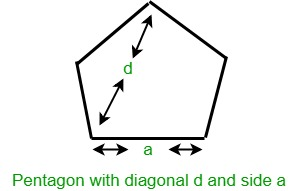# Calculate area of pentagon with given diagonal

Given an integer d which is the length of the diagonal of a pentagon, the task is to find the area of that pentagon.Examples:

Input: d = 5
Output: 16.4291

Input: d = 10
Output: 65.7164

## Recommended: Please try your approach on {IDE} first, before moving on to the solution.

Approach: Pentagon is a regular polygon having five equal sides and all equal angles. The interior angles of pentagon are of 108 degrees each and the sum of all angles of a pentagon is 540 degrees. If d is the diagonal of the pentagon then it’s area is given by:Below is the implementation of the above approach:

## C++

 // C++ program to find the area of   // Pentagon with given diagonal  #include  using namespace std;     // Function to return the area of the  // pentagon with diagonal d  float pentagonArea(float d)  {      float area;         // Formula to find area      area = (d * d * (-5 + sqrt(45)) * sqrt(sqrt(20) + 5)) / 8;         return area;  }     // Driver code  int main()  {      float d = 5;      cout << pentagonArea(d);      return 0;  }

## Java

 // Java program to find the area of   // Pentagon with given diagonal  import java.text.*;  class GFG{  // Function to return the area of the  // pentagon with diagonal d  static double pentagonArea(double d)  {      double area;         // Formula to find area      area = (d * d * (-5 + Math.sqrt(45)) * Math.sqrt(Math.sqrt(20) + 5)) / 8;         return area;  }     // Driver code  public static void main(String[] args)  {      double d = 5;      DecimalFormat dec = new DecimalFormat("#0.0000");      System.out.println(dec.format(pentagonArea(d)));  }  }  // This code is contributed by mits

## Python3

 # Python3 program to find the area of   # Pentagon with given diagonal      # from math lib import sqrt() method  from math import sqrt     # Function to return the area of the   # pentagon with diagonal d   def pentagonArea(d) :         # Formula to find area       area = (d * d * (-5 + sqrt(45)) * sqrt(sqrt(20) + 5)) / 8        return round(area , 4)         # Driver code   if __name__ == "__main__" :         d = 5     print(pentagonArea(d))     # This code is contributed by Ryuga

## C#

 // C# program to find the area of   // Pentagon with given diagonal  using System;     class GFG{  // Function to return the area of the  // pentagon with diagonal d  static double pentagonArea(double d)  {      double area;         // Formula to find area      area = (d * d * (-5 + Math.Sqrt(45)) * Math.Sqrt(Math.Sqrt(20) + 5)) / 8;         return area;  }     // Driver code  public static void Main()  {      double d = 5;      Console.WriteLine("{0:F4}",pentagonArea(d));  }  }  // This code is contributed by mits

## PHP

 

Output:

16.4291
`

Attention reader! Don’t stop learning now. Get hold of all the important DSA concepts with the DSA Self Paced Course at a student-friendly price and become industry ready.

My Personal Notes arrow_drop_upCheck out this Author's contributed articles.

If you like GeeksforGeeks and would like to contribute, you can also write an article using contribute.geeksforgeeks.org or mail your article to contribute@geeksforgeeks.org. See your article appearing on the GeeksforGeeks main page and help other Geeks.

Please Improve this article if you find anything incorrect by clicking on the "Improve Article" button below.